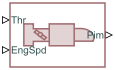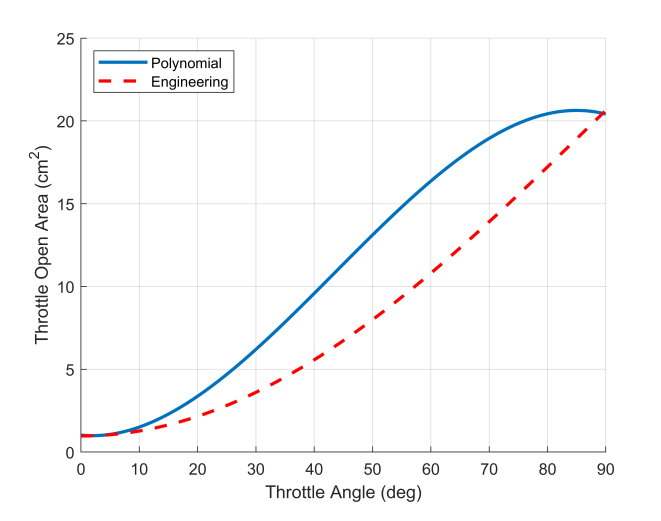# Air Intake

Combustion cylinder air supply intake

Since R2022a

•Libraries:
Simscape / Driveline / Engines & Motors / Engine Subcomponents

## Description

The Air Intake block represents an abstract air intake system that computes lumped-parameter air flow dynamics. This block is suitable for internal combustion engines. The block computes the intake manifold pressure from the throttle and engine speed.

### Equations

The Air Intake block computes the intake manifold pressure as

`$\frac{d}{dt}{P}_{im}=\frac{{R}_{air}{T}_{amb}}{{V}_{im}}\left({\stackrel{˙}{m}}_{thr}-{\stackrel{˙}{m}}_{cyl}\right),$`

where

• Rair is the Dry air specific gas constant parameter.

• Tamb is the Ambient temperature parameter.

• Vim is the Intake manifold volume parameter.

The block calculates the combustion cylinder mass flow rate, cyl, as

`${\stackrel{˙}{m}}_{cyl}=\frac{\omega {V}_{d}{P}_{im}}{2{R}_{air}{T}_{amb}},$`

which is a time derivative formulation of the ideal gas law. ω is the engine speed the block receives from port EngSpd, and Vd is the Engine displacement volume parameter.

The block calculates the throttle mass flow rate, thr as

depending on the flow conditions. In this equation, Sthr is the area of the throttle opening, and Pamb is the ambient pressure. The block defines the equation constants as

`$\begin{array}{l}{c}_{a}=\frac{\kappa +1}{\kappa }\\ {c}_{b}=2}{\kappa }\\ {c}_{1}=\frac{\sqrt{{c}_{2}\left({\Pi }_{lam}^{{c}_{b}}-{\Pi }_{lam}^{{c}_{a}}\right)}}{1-{\Pi }_{lam}}\\ {c}_{2}=\frac{2\kappa }{{R}_{air}\left(\kappa -1\right)}\\ {c}_{3}=\sqrt{\frac{\kappa }{{R}_{air}}{\Pi }_{cr}^{{c}_{a}}}\end{array}$`

where

• κ is the Dry air specific heat ratio parameter.

• Πlam is the laminar flow threshold constant.

• Πcr is the critical pressure ratio.

The block finds Πcr as

`${\Pi }_{cr}={\left(\frac{2}{\kappa +1}\right)}^{\frac{\kappa }{\kappa -1}}.$`

Throttle Open Area

The block treats the throttle open area, Sthr, as a third-order polynomial that functions with respect to the throttle angle, θ. This method simplifies the block computation to improve performance. The block computes the throttle open area as

`${S}_{thr}\left(\theta \right)={k}_{0}+{k}_{1}\theta +{k}_{2}{\theta }^{2}+{k}_{3}{\theta }^{3},$`

where the block derives the coefficients, ki, using these quantities:

• DplateThrottle plate diameter parameter

• ArestThrottle rest angle parameter

• Apeak — 85°

• SleakThrottle leakage area parameter

The throttle open area uses these constraints:

`$\begin{array}{c}{S}_{thr}\left(0\right)={S}_{eng}\left(0\right)\\ {S}_{thr}\left(\frac{\pi }{2}\right)={S}_{eng}\left({A}_{peak}\right)\\ \frac{d}{d\theta }{S}_{thr}\left({A}_{rest}\right)=\frac{d}{d\theta }{S}_{eng}\left({A}_{rest}\right)\\ {\frac{d}{d\theta }}_{thr}{S}_{thr}\left({A}_{peak}\right)=0\end{array}$`

where Seng is the engineering formula for the throttle open area, such that

`${S}_{eng}\left(\theta \right)=\pi {\left(\frac{{D}_{plate}}{2}\right)}^{2}\left(1-\frac{\mathrm{cos}\left(\theta \right)}{\mathrm{cos}\left({A}_{rest}\right)}\right)+{S}_{leak}.$`

This figure shows a comparison of the polynomial result and the engineering result.## Ports

### Input

expand all

Physical signal input associated with the normalized throttle command, where `0` represents a fully closed throttle, and `1` represents a fully open throttle. The block maps this input to a throttle-open angle between `0` and `90` degrees.

Physical signal input associated with the rotational speed, in rad/s.

### Output

expand all

Physical signal input associated with the intake manifold, in kPa.

## Parameters

expand all

Specific gas constant of dry air.

Specific heat ratio of dry air.

Pressure of the air entering the intake.

Temperature of the air entering the intake.

Radial distance from the resting throttle position to `0` degrees.

Diameter of the butterfly valve disc in the throttle body.

Cumulative area of throttle leakage.

Volumetric capacity of the intake manifold.

Combined displacement of all engine pistons.

Time constant associated with the response rate of the engine to the throttle command.

## Version History

Introduced in R2022a